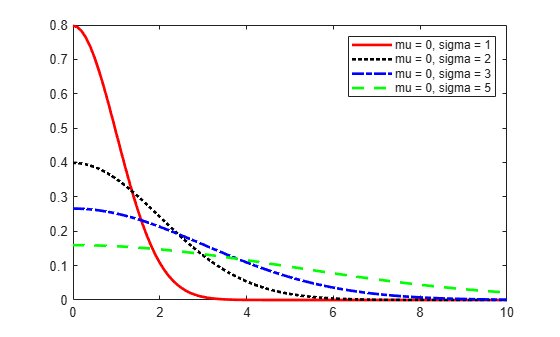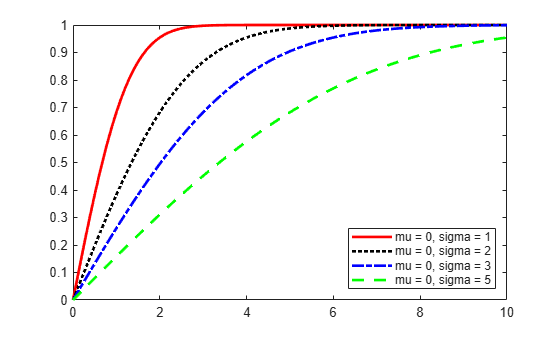## Half-Normal Distribution

### Overview

The half-normal distribution is a special case of the folded normal and truncated normal distributions. Some applications of the half-normal distribution include modeling measurement data and lifetime data.

### Parameters

The half-normal distribution uses the following parameters:

ParameterDescription
$-\infty <\mu <\infty$Location parameter
$\sigma \ge 0$Scale parameter

The support for the half-normal distribution is xμ.

Use `makedist` with specified parameter values to create a half-normal probability distribution object `HalfNormalDistribution`. Use `fitdist` to fit a half-normal probability distribution object to sample data. Use `mle` to estimate the half-normal distribution parameter values from sample data without creating a probability distribution object. For more information about working with probability distributions, see Working with Probability Distributions.

The Statistics and Machine Learning Toolbox™ implementation of the half-normal distribution assumes a fixed value for the location parameter μ. Therefore, neither `fitdist` nor `mle` estimates the value of the parameter μ when fitting a half-normal distribution to sample data. You can specify a value for the μ parameter by using the name-value pair argument `'mu'`. The default value for the `'mu'` argument is 0 in both `fitdist` and `mle`.

### Probability Density Function

The probability density function (pdf) of the half-normal distribution is

`$y=f\left(x|\mu ,\sigma \right)=\sqrt{\frac{2}{\pi }}\frac{1}{\sigma }{e}^{-\frac{1}{2}{\left(\frac{x-\mu }{\sigma }\right)}^{2}}\text{\hspace{0.17em}};\text{\hspace{0.17em}}x\ge \mu \text{\hspace{0.17em}},$`

where μ is the location parameter and σ is the scale parameter. If xμ, then the pdf is undefined.

To compute the pdf of the half-normal distribution, create a `HalfNormalDistribution` probability distribution object using `fitdist` or `makedist`, then use the `pdf` method to work with the object.

#### PDF of Half-Normal Probability Distribution

This example shows how changing the values of the `mu` and `sigma` parameters alters the shape of the pdf.

Create four probability distribution objects with different parameters.

```pd1 = makedist('HalfNormal'); pd2 = makedist('HalfNormal','mu',0,'sigma',2); pd3 = makedist('HalfNormal','mu',0,'sigma',3); pd4 = makedist('HalfNormal','mu',0,'sigma',5);```

Compute the probability density functions (pdfs) of each distribution.

```x = 0:0.1:10; pdf1 = pdf(pd1,x); pdf2 = pdf(pd2,x); pdf3 = pdf(pd3,x); pdf4 = pdf(pd4,x);```

Plot the pdfs on the same figure.

```figure; plot(x,pdf1,'r','LineWidth',2) hold on; plot(x,pdf2,'k:','LineWidth',2); plot(x,pdf3,'b-.','LineWidth',2); plot(x,pdf4,'g--','LineWidth',2); legend({'mu = 0, sigma = 1','mu = 0, sigma = 2',... 'mu = 0, sigma = 3','mu = 0, sigma = 5'},'Location','NE'); hold off;```As `sigma` increases, the curve flattens and the peak value becomes smaller.

### Cumulative Distribution Function

The cumulative distribution function (cdf) of the half-normal distribution is

`$y=F\left(x\right)=erf\left(\frac{x-\mu }{\sqrt{2}\sigma }\right)=2\Phi \left(\frac{x-\mu }{\sigma }\right)-1\text{\hspace{0.17em}};\text{\hspace{0.17em}}x\ge \mu \text{\hspace{0.17em}},$`

where μ is the location parameter, σ is the scale parameter, erf(•) is the error function, and Φ(•) is the cdf of the standard normal distribution. If xμ, then the cdf is undefined.

To compute the cdf of the half-normal distribution, create a `HalfNormalDistribution` probability distribution object using `fitdist` or `makedist`, then use the `cdf` method to work with the object.

#### CDF of Half-Normal Probability Distribution

This example shows how changing the values of the `mu` and `sigma` parameters alters the shape of the cdf.

Create four probability distribution objects with different parameters.

```pd1 = makedist('HalfNormal'); pd2 = makedist('HalfNormal','mu',0,'sigma',2); pd3 = makedist('HalfNormal','mu',0,'sigma',3); pd4 = makedist('HalfNormal','mu',0,'sigma',5);```

Compute the cumulative distribution functions (cdfs) for each probability distribution.

```x = 0:0.1:10; cdf1 = cdf(pd1,x); cdf2 = cdf(pd2,x); cdf3 = cdf(pd3,x); cdf4 = cdf(pd4,x);```

Plot all four cdfs on the same figure.

```figure; plot(x,cdf1,'r','LineWidth',2) hold on; plot(x,cdf2,'k:','LineWidth',2); plot(x,cdf3,'b-.','LineWidth',2); plot(x,cdf4,'g--','LineWidth',2); legend({'mu = 0, sigma = 1','mu = 0, sigma = 2',... 'mu = 0, sigma = 3','mu = 0, sigma = 5'},'Location','SE'); hold off;```As `sigma` increases, the curve of the cdf flattens.

### Descriptive Statistics

The mean of the half-normal distribution is

`$\text{mean}=\mu +\sigma \sqrt{\frac{2}{\pi }\text{\hspace{0.17em}},}$`

where μ is the location parameter and σ is the scale parameter.

The variance of the half-normal distribution is

`$\mathrm{var}={\sigma }^{2}\left(1-\frac{2}{\pi }\right)\text{\hspace{0.17em}},$`

where σ is the scale parameter.

### Relationship to Other Distributions

If a random variable `Z` has a standard normal distribution with a mean μ equal to zero and standard deviation σ equal to one, then $X=\mu +\sigma |Z|$ has a half-normal distribution with parameters μ and σ.

 Cooray, K. and M.M.A. Ananda. “A Generalization of the Half-Normal Distribution with Applications to Lifetime Data.” Communications in Statistics – Theory and Methods. Vol. 37, Number 9, 2008, pp. 1323–1337.

 Pewsey, A. “Large-Sample Inference for the General Half-Normal Distribution.” Communications in Statistics – Theory and Methods. Vol. 31, Number 7, 2002, pp. 1045–1054.